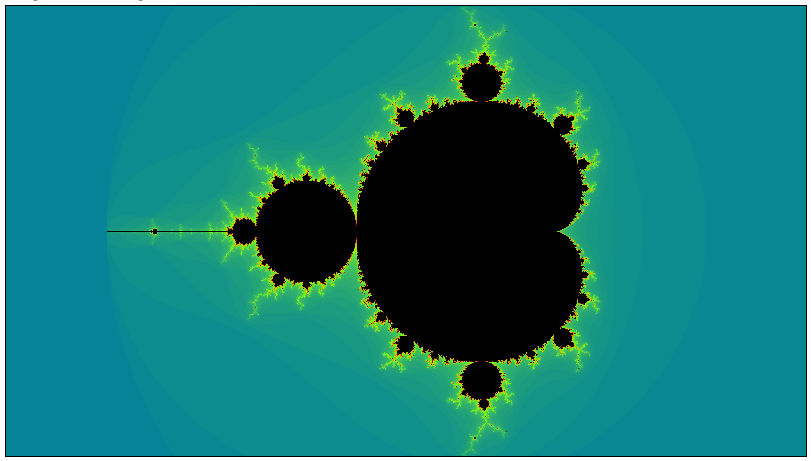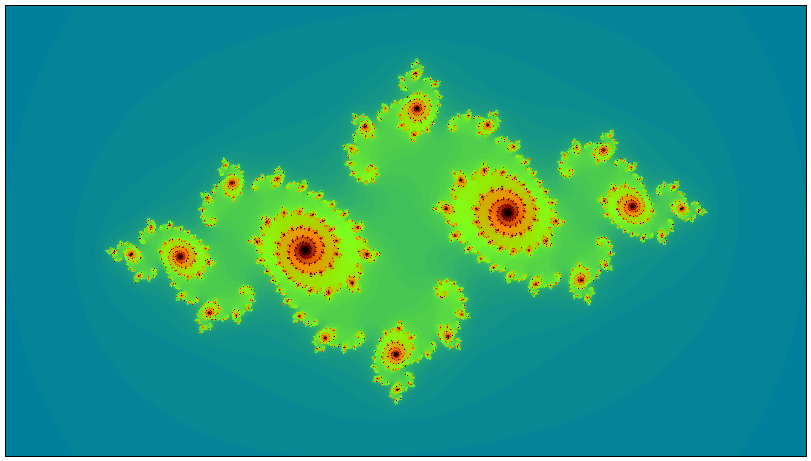# Plotting Fractals in WebAssembly

Previous   Next
Top 2: Initial Implementation

# 1: Plotting Fractals

To create any sort of fractal image, it is necessary to repeatedly evaluate a function that takes a complex number as input and gives another complex number as output. This output value is then fed back into the original equation and a new value is generated.

In order to calculate the colour of one pixel, we must repeat this iterative calculation hundreds, if not thousands of times.

As you can imagine, this type of coding is completely CPU-bound, and is therefore ideally suited for implementation in WebAssembly.

Very conveniently, the value of any one pixel in a fractal image is quite unrelated to the value of any neighbouring pixels; therefore, the calculation of the overall image is said to be “embarrassingly parallel”. That is, we can split up the calculation across multiple, independent instances of the same program.

This greatly improves runtime efficiency, and will also be demonstrated here.

## The Mandelbrot SetThe Mandelbrot Set is created as follows:

1. First, decide on two arbitrary limits:
• What is the maximum number of times we will iterate the calculation before giving up? This value is referred to as `max_iters` and a reasonable starting value is `1000`
• Each time we iterate the calculation, we get out a number that might well start to grow. How big should we allow this number to become before stopping (since it’s only going to continue growing). This limit is called `bailout` and is typically set to `4`
2. Using this equation:For any given starting point `c` on the complex plane and with Z0 = 0, pass these values through the above equation to derive the new value Z1.

Repeatedly derive Zn+1 by passing Zn into the equation (counting the number of iterations) until either `|Z| > bailout` or we hit the iteration limit defined by `max_iters`

3. Colour each pixel according to the number of times the equation is iterated. Any pixel whose iteration value hits the `max_iters` limit is arbitrarily coloured black. The other pixels can be coloured according whatever aesthetically pleasing colour scheme you choose

4. Repeat steps 2 and 3 for every pixel in the image

## Julia Sets

For every point on the Mandelbrot Set, there is a corresponding Julia Set. This means that there are an infinite number of possible Julia Sets, and the one shown here corresponds to the location (-0.755, -0.19) on the Mandelbrot Set.